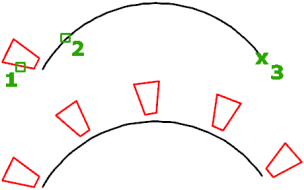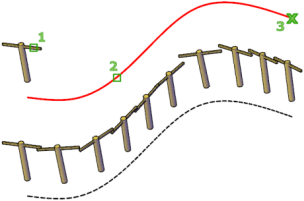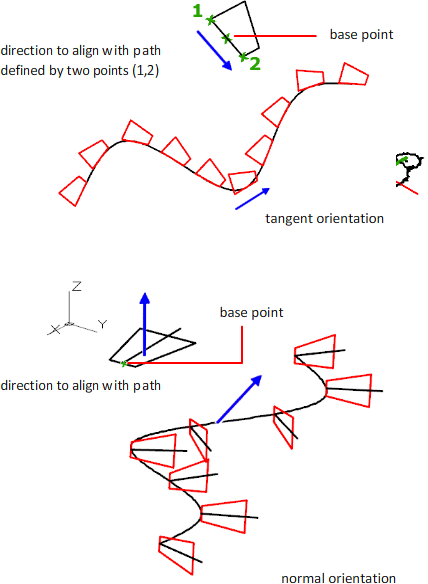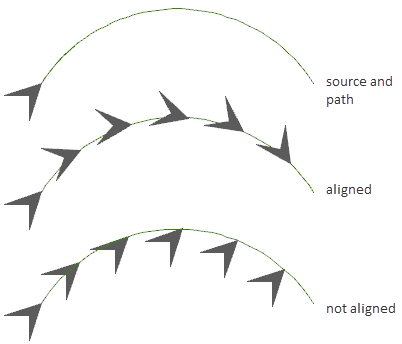# ARRAYPATH (Command)

Evenly distributes object copies along a path or a portion of a path.

Find

The path can be a line, polyline, 3D polyline, spline, helix, arc, circle, or ellipse.2D path array3D path array

This command is equivalent to the Path option in ARRAY. The DELOBJ system variable controls whether the source objects of the array are deleted or retained after the array is created.

Note: The 3D objects used in the example cannot be created in AutoCAD LT.

The following prompts are displayed.

### Select objects

Select the objects to use in the array.

### Path curve

Specifies the object to use for the path of the array. Select a line, polyline, 3D polyline, spline, helix, arc, circle, or ellipse.

### Associative

Specifies whether an arrayed object is created or whether the unassociated copies of the selected object are created.

Yes
Creates array items in a single array object, similar to a block. With an associative array, you can quickly propagate changes throughout the array by editing the properties and source objects.
No
Creates array items as independent objects. Changes to one item do not affect the other items.

### Method

Controls how to distribute items along the path.

Divide
Distributes a specified number of items evenly along the length of the path.
Measure
Distributes items along the path at specified intervals.

### Base point

Defines the base point of the array. Items in path arrays are positioned relative to the base point.

Base point

Specifies a base point for positioning the items in the array relative to the start of the path curve.

Key point

For associative arrays, specifies a valid constraint (or key point) on the source objects to align with the path. If you edit the source objects or path of the resulting array, the base point of the array remains coincident with the key point of the source objects.

### Tangent direction

Specifies how the arrayed items are aligned relative to the starting direction of the path.

2 points
Specifies two points that represent the tangency of the arrayed items relative to the path. The vector of the two points establishes the tangency of the first item in the array. The Align Items setting controls whether the other items in the array maintain a tangent or parallel orientation.
Normal
Orients the Z direction of the first item with the starting direction of the path curve.### Items

Depending on the Method setting, specifies the number of items or the distance between items.

Number of items along path
(Available when Method equals Divide) Using a value or expression, specifies how many items are in the array.
Distance between items along path
(Available when Method equals Measure) Using a value or expression, specifies the distance between arrayed items.

By default, the array is populated with the maximum number of items that fill the path using the distance entered. You can specify a smaller number of items if desired. You can also turn on Fill Entire Path so that the number of items is adjusted if the length of the path changes.

### Rows

Specifies the number of rows in the array, the distance between them, and the incremental elevation between row.

Number of rows

Sets the number of rows.

Distance between rows
Specifies the distance between each row, measured from equivalent locations on each object.
Total
Specifies the total distance between the start and end row, measured from equivalent locations on the start and end objects.
Incrementing elevation
Sets the increasing or decreasing elevation for each subsequent row.
Expression

Derives the value based on a mathematical formula or equation.

### Levels

Levels in an array refers to extending the row and column pattern of an array in the Z-axis direction.

Number of levels
Specifies the number of 3D levels in the array.
Distance between levels
Specifies the distance between the 3D levels.
Total

Specifies the total distance between the first and last levels.

Expression

Derives a value using a mathematical formula or equation.

### Align items

Specifies whether to align each item to be tangent to the path direction. Alignment is relative to the first item’s orientation.### Z direction

Controls whether to maintain the items’ original Z direction or to naturally bank the items along a 3D path.

### Exit

Ends the command.